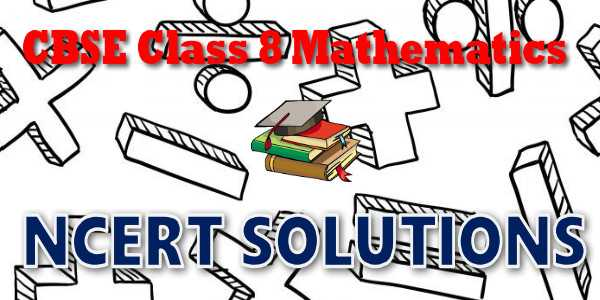# CBSE - Class 08 - Mathematics - Algebraic Expressions and Identities - NCERT Solutions## NEET-UG 2021

10,000+ Daily Practice Questions

(only at ₹ 299/- in myCBSEguide App)

## NCERT Solutions for Mathematics Algebraic Expressions and Identities## myCBSEguide App

Complete Guide for CBSE Students

NCERT Solutions, NCERT Exemplars, Revison Notes, Free Videos, CBSE Papers, MCQ Tests & more.

# NCERT solutions for class 8 Mathematics Algebra Expression and Identities## NCERT Class 8 Mathematics Chapter-wise Solutions

Maths Part I

• Chapter 1 - Rational Numbers
• Chapter 2 - Linear Equations in One Variable
• Chapter 3 - Understanding Quadrilaterals
• Chapter 4 - Practical Geometry
• Chapter 5 - Data Handling
• Chapter 6 - Squares and Square Roots
• Chapter 7 - Cubes and Cube Roots
• Chapter 8 - Comparing Quantities
• Chapter 9 - Algebraic Expressions and Identities
• Chapter 10 - Visualising Solid Shapes
• Chapter 11 - Mensuration
• Chapter 12 - Exponents and Powers
• Chapter 13 - Direct and Inverse Proportions
• Chapter 14 - Factorisation
• Chapter 15 - Introduction to Graphs
• Chapter 16 - Playing with Numbers

## CBSE class 8th Mathematics have one books. Each book has chapters and topics.

• NCERT Mathematics Book Class 8 Part-1

Here is the list of topics covered under each chapter of class 8 Mathematics NCERT text book.

• Exercise 1.1
• Exercise 1.2

• Exercise 2.1
• Exercise 2.2
• Exercise 2.3
• Exercise 2.4
• Exercise 2.5
• Exercise 2.6

• Exercise 3.1
• Exercise 3.2
• Exercise 3.3
• Exercise 3.4

• Exercise 4.1
• Exercise 4.2
• Exercise 4.3
• Exercise 4.4
• Exercise 4.5

• Exercise 5.1
• Exercise 5.2
• Exercise 5.3

• Exercise 6.1
• Exercise 6.2
• Exercise 6.3
• Exercise 6.4

• Exercise 7.1
• Exercise 7.2

• Exercise 8.1
• Exercise 8.2
• Exercise 8.3

• Exercise 9.1
• Exercise 9.2
• Exercise 9.3
• Exercise 9.4
• Exercise 9.5

### 10. Visualising Solid Shapes

• Exercise 10.1
• Exercise 10.2
• Exercise 10.3

### 11. Mensuration

• Exercise 11.1
• Exercise 11.2
• Exercise 11.3
• Exercise 11.4

### 12. Exponents and Powers

• Exercise 12.1
• Exercise 12.2

### 13. Direct and Inverse Proportions

• Exercise 13.1
• Exercise 13.2

### 14. Factorisation

• Exercise 14.1
• Exercise 14.2
• Exercise 14.3
• Exercise 14.4

### 15. Introduction to Graphs

• Exercise 15.1
• Exercise 15.2
• Exercise 15.3

### 16.Playing with Numbers

• Exercise 16.1
• Exercise 16.2

## NCERT Solutions Chapter 9 Algebraic Expressions and Identities

In earlier classes, we have already become familiar with what algebraic expressions
(or simply expressions) are. Examples of expressions are:
x + 3, 2y – 5, 3x2, 4xy + 7 etc.
You can form many more expressions.As you know expressions are formed from variables and constants. The expression 2y – 5 is formed from the variable yand constants 2 and 5. The expression 4xy + 7 is formed from variables x and y and constants 4 and 7. We know that, the value of y in the expression, 2y – 5, may be anything. It can be The value of an expression changes with the value chosen for the variables it contains. Thus as y takes on different values, the value of 2y – 5 goes on changing. When y = 2, 2y – 5 = 2(2) – 5 = –1; when y = 0, 2y – 5 = 2 × 0 –5 = –5, etc. Find the value of the expression 2y – 5 for the other given values of y

## NCERT Solutions for Class 8th Mathematics

NCERT Solutions Class 8 Mathematics PDF (Download) Free from myCBSEguide app and myCBSEguide website. Ncert solution class 8 Mathematics includes text book solutions from  part . NCERT Solutions for CBSE Class 8 Mathematics have total 16 chapters. Class 8 Mathematics ncert Solutions in pdf for free Download are given in this website. Ncert Mathematics class 8 solutions PDF and Mathematics ncert class 8 PDF solutions with latest modifications and as per the latest CBSE syllabus are only available in myCBSEguide.

## NCERT Solutions for Class 5

NCERT Solutions for Class 4

## NCERT Solutions for Class 3## myCBSEguide

Trusted by 1 Crore+ Students

#### CBSE Test Generator

Create papers in minutes

Print with your name & Logo

3 Lakhs+ Questions

Solutions Included

Based on CBSE Blueprint

Best fit for Schools & Tutors

#### Work from Home

• Work from home with us
• Create questions or review them from home

No software required, no contract to sign. Simply apply as teacher, take eligibility test and start working with us. Required desktop or laptop with internet connection